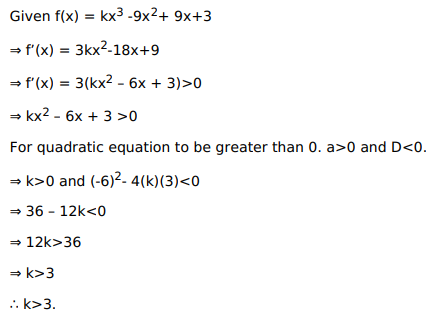# Solve this following

Question:

Mark $(\sqrt{)}$ against the correct answer in the following:

If $f(\mathrm{x})=\mathrm{kx}^{3}-9 \mathrm{x}^{2}+9 \mathrm{x}+3$ is increasing for every real number $\mathrm{x}$, then

A. $k>3$

B. $k \geq 3$

C. $k<3$

D. $k \leq 3$

Solution: